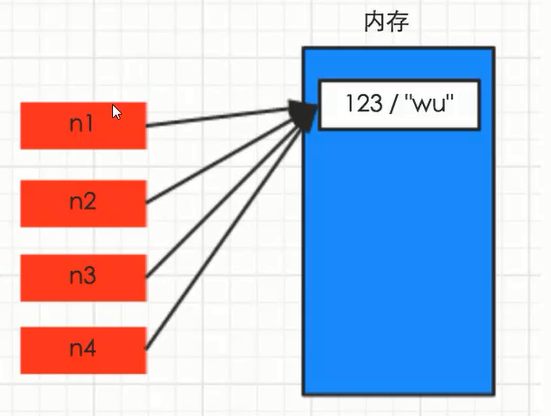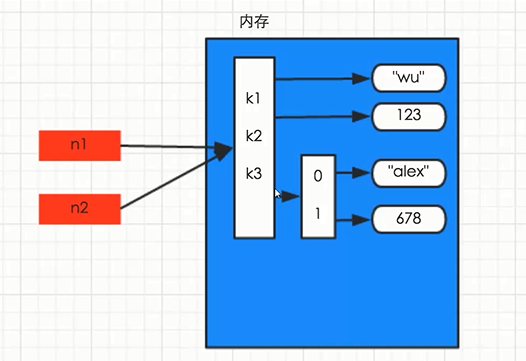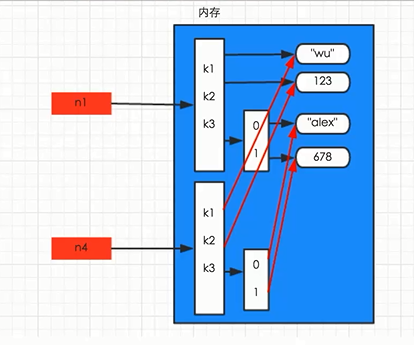# python中的赋值与深浅拷贝

Python当中对于拷贝，分为两种类型。一种是数字和字符串，另一种就是列表、元组、字典等其他类型了。

# 一、数字和字符串的拷贝

## 1、赋值

a1 = 123123
a2 = 123123
# a2 = a1  # 赋值
print(id(a1))  # 通过id()函数来打印变量在内存当中的地址
print(id(a2))

1959780298352
1959780298352　

在以上代码块当中，a2与a1所赋的值是一样的，都是数字123123。因为python有一个重用机制，对于同一个数字，python并不会开辟一块新的内存空间，而是维护同一块内存地址，只是将该数字对应的内存地址的引用赋值给变量a1和a2。所以根据输出结果，a1和a2其实对应的是同一块内存地址，只是两个不同的引用罢了。同样的，对于a2 = a1，其实效果等同于“a1 = 123123; a2 = 123123”，它也就是将a1指向123123的引用赋值给a2。字符串跟数字的原理雷同，如果把123123改成“abcabc”也是一样的。

## 2、浅拷贝

import copy  # 使用浅拷贝需要导入copy模块

a1 = 123123
a3 = copy.copy(a1)  # 使用copy模块里的copy()函数就是浅拷贝了
print(id(a1))
print(id(a3))

35233168
35233168

通过使用copy模块里的copy()函数来进行浅拷贝，把a1拷贝一份赋值给a3，查看输出结果发现，a1和a3的内存地址还是一样。

## 3、深拷贝

import copy

a1 = 123123
a4 = copy.deepcopy(a1)  # 深拷贝是用copy模块里的deepcopy()函数
print(id(a1))
print(id(a4))

31432080
31432080

查看结果发现，对于深拷贝，数字和字符串在内存当中用的也是同一块地址。# 二、字典、列表、元组等其他类型的拷贝

## 1、赋值

n1 = {"k1": "wu", "k2": 123, "k3": ["alex", 678]}
n2 = n1  # 赋值
print(id(n1))
print(id(n2))

2235551677536
2235551677536

我们的栗子当中用了一个字典n1，字典里面嵌套了一个列表，当我们把n1赋值给n2时，内存地址并没有发生变化，因为其实它也是只是把n1的引用拿过来赋值给n2而已。（我们用了一个字典来举例，其他类型也是一样的）## 2、浅拷贝

import copy

n1 = {"k1": "wu", "k2": 123, "k3": ["alex", 678]}
n3 = copy.copy(n1)  # 浅拷贝
print("第一层字典的内存地址：")
print(id(n1))
print(id(n3))
print("第二层嵌套的列表的内存地址：")
print(id(n1["k3"]))
print(id(n3["k3"]))

第一层字典的内存地址：
6516024
6516096

36995720
36995720

通过以上结果可以看出，进行浅拷贝时，我们的字典第一层n1和n3指向的内存地址已经改变了，但是对于第二层里的列表并没有拷贝，它的内存地址还是一样的。原理如下图：结论：所以对于浅拷贝，字典、列表、元组等类型，它们只拷贝第一层地址。

## 3、深拷贝

import copy

n1 = {"k1": "wu", "k2": 123, "k3": ["alex", 678]}
n4 = copy.deepcopy(n1)  # 深拷贝
print("第一层字典的内存地址：")
print(id(n1))
print(id(n4))
print("第二层嵌套的列表的内存地址：")
print(id(n1["k3"]))
print(id(n4["k3"]))

第一层字典的内存地址：
31157560
35463600

35947144
35947336

通过以上结果发现，进行深拷贝时，字典里面的第一层和里面嵌套的地址都已经变了。对于深拷贝，它会拷贝多层，将第二层的列表也拷贝一份，如果还有第三层嵌套，那么第三层的也会拷贝，但是对于里面的最小元素，比如数字和字符串，这里就是“wu”，123，“alex”，678之类的，按照python的机制，它们会共同指向同一个位置，它的内存地址是不会变的。原理如下图：import copy

dic = {
"cpu": [80, ],
"mem": [80, ],
"disk": [80, ]
}
# 定义了一个字典，存储服务器信息。
print('before', dic)
new_dic = copy.copy(dic)
new_dic['cpu'] = 50  # 更新cpu为50
print(dic)
print(new_dic)

before {'cpu': , 'mem': , 'disk': }
{'cpu': , 'mem': , 'disk': }
{'cpu': , 'mem': , 'disk': }

import copy

dic = {
"cpu": [80, ],
"mem": [80, ],
"disk": [80, ]
}
print('before', dic)
new_dic = copy.deepcopy(dic)
new_dic['cpu'] = 50
print(dic)
print(new_dic)

before {'cpu': , 'mem': , 'disk': }
{'cpu': , 'mem': , 'disk': }
{'cpu': , 'mem': , 'disk': }

注：本文是根据老男孩课程内容整理而成的，图片也是出自武sir的，因为原文我找不到了，所以下面贴一下武sir的博客地址。本文仅供个人笔记使用，如果有侵犯，请联系我，我立即撤销。

posted @ 2018-04-09 15:33  doublexi  阅读(6637)  评论(4编辑  收藏  举报